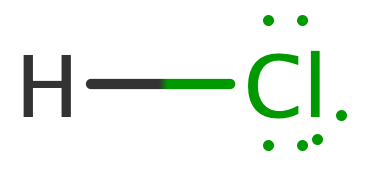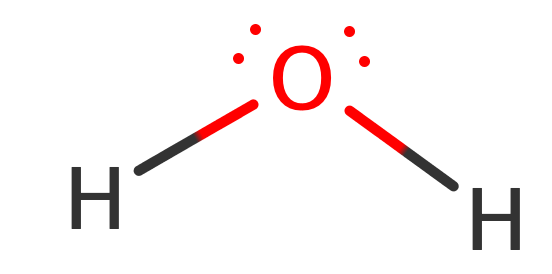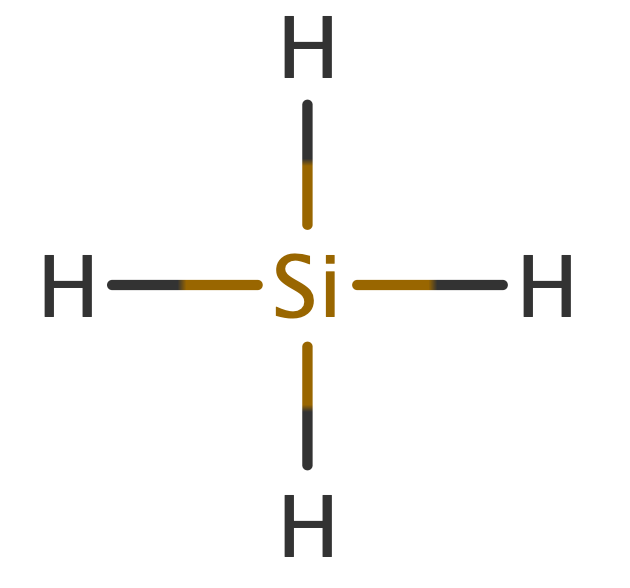# Problem: Arrange each of the following sets of compounds in order of increasing boiling point temperature:(a) HCl, H2O, SiH4

###### FREE Expert Solution

HCl

total valence e- = 8 e-

Lewis structure:ΔEN = 3.0 – 2.2 = 0.8 polar covalent bond

dipole-dipole forces

H2O

total valence e- = 8 e-

Lewis Structure:ΔEN = 3.5 – 2.2 = 1.3 polar covalent bond

O connected to H hydrogen-bonding

SiH4

total valence e- = 8 e-

Lewis StructureΔEN = 2.2 – 1.8 = 0.4 polar covalent bond

no net dipole London dispersion forces

81% (190 ratings)###### Problem Details

Arrange each of the following sets of compounds in order of increasing boiling point temperature:

(a) HCl, H2O, SiH4

Frequently Asked Questions

What scientific concept do you need to know in order to solve this problem?

Our tutors have indicated that to solve this problem you will need to apply the Intermolecular Forces and Physical Properties concept. If you need more Intermolecular Forces and Physical Properties practice, you can also practice Intermolecular Forces and Physical Properties practice problems.

What professor is this problem relevant for?

Based on our data, we think this problem is relevant for Professor Yang's class at FSU.

What textbook is this problem found in?

Our data indicates that this problem or a close variation was asked in Chemistry - OpenStax 2015th Edition. You can also practice Chemistry - OpenStax 2015th Edition practice problems.